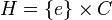# Characteristic not implies characteristic-isomorph-free in finite

This article gives the statement and possibly, proof, of a non-implication relation between two subgroup properties. That is, it states that every subgroup satisfying the first subgroup property (i.e., characteristic subgroup) need not satisfy the second subgroup property (i.e., characteristic-isomorph-free subgroup)
View a complete list of subgroup property non-implications | View a complete list of subgroup property implications
EXPLORE EXAMPLES YOURSELF: View examples of subgroups satisfying property characteristic subgroup but not characteristic-isomorph-free subgroup|View examples of subgroups satisfying property characteristic subgroup and characteristic-isomorph-free subgroup

## Statement

We can find a group$G$ (in fact, we can choose$G$ to be a finite group) and characteristic subgroups$H,K$ of$G$ that are not isomorphic to each other but distinct.

## Proof

### Example of the infinite cyclic group

Let$\mathbb{Z}$ be the infinite cyclic group: the group of integers under addition. Then,$n\mathbb{Z}$ is a characteristic subgroup of$\mathbb{Z}$ for any$n$, and all the$n\mathbb{Z}$ are isomorphic for$n$ a nonnegative integer. Thus, there are distinct characteristic subgroups that are isomorphic.

### Example of a finite solvable group

Further information: symmetric group:S3

Let$A$ be a nontrivial metabelian group that is also a centerless group, with derived subgroup$B$. Let$C$ be a group isomorphic to$B$. Then, in the direct product$G := A \times C$, we have:

• The subgroup$H = \{ e \} \times C$ equals the center of$A \times C$, hence is characteristic.
• The subgroup$K = A \times \{ e \}$ equals the derived subgroup of$A \times C$, hence is characteristic.

Thus, we have two distinct characteristic subgroups that are isomorphic.

A particular case of this is where$A$ is the symmetric group on three letters. In this case,$B$ is A3 in S3 and$C$ is cyclic of order three.

### Example of a finite nilpotent group

There are groups of order$p^5$ with characteristic maximal subgroups that are isomorphic. For instance, the group with GAP Group ID$(243,5)$ has three pairwise isomorphic characteristic subgroups (each with group ID$(81,3)$).